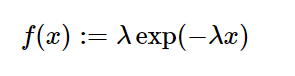Related Articles
sympy.stats.Exponential() in python
• Last Updated : 05 Jun, 2020

With the help of `sympy.stats.Exponential()` method, we can get the continuous random variable representing the exponential distribution.Syntax : `sympy.stats.Exponential(name, rate)`
Return : Return continuous random variable.

Example #1 :
In this example we can see that by using `sympy.stats.Exponential()` method, we are able to get the continuous random variable which represents the Exponential distribution by using this method.

 `# Import sympy and Exponential``from` `sympy.stats ``import` `Exponential, density``from` `sympy ``import` `Symbol`` ` `rate ``=` `Symbol(``"rate"``, integer ``=` `True``, positive ``=` `True``)``z ``=` `Symbol(``"z"``)`` ` `# Using sympy.stats.Exponential() method``X ``=` `Exponential(``"x"``, rate)``gfg ``=` `density(X)(z)`` ` `pprint(gfg)`

Output :

-rate*z
rate*e

Example #2 :

 `# Import sympy and Exponential``from` `sympy.stats ``import` `Exponential, density``from` `sympy ``import` `Symbol`` ` `rate ``=` `5``z ``=` `0.5`` ` `# Using sympy.stats.Exponential() method``X ``=` `Exponential(``"x"``, rate)``gfg ``=` `density(X)(z)`` ` `pprint(gfg)`

Output :

0.410424993119494

Attention geek! Strengthen your foundations with the Python Programming Foundation Course and learn the basics.

To begin with, your interview preparations Enhance your Data Structures concepts with the Python DS Course. And to begin with your Machine Learning Journey, join the Machine Learning – Basic Level Course

My Personal Notes arrow_drop_up